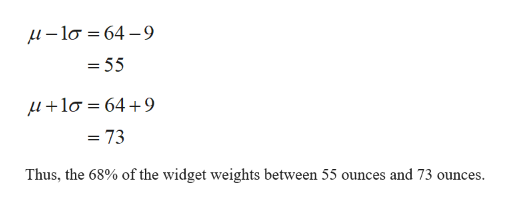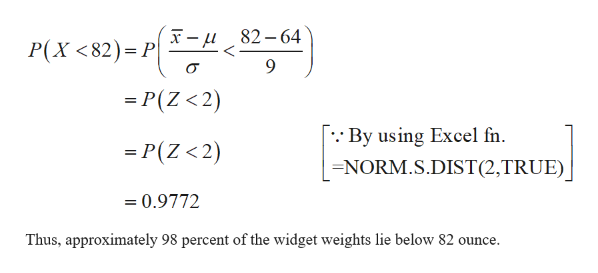# The Acme Company manufactures widgets. The distribution of widget weights is bell-shaped. The widget weights have a mean of 64 ounces and a standard deviation of 9 ounces.a) 68% of the widget weights lie between  and b) What percentage of the widget weights lie between 37 and 73 ounces?  %c) What percentage of the widget weights lie below 82 ?  %

Question
2 views

The Acme Company manufactures widgets. The distribution of widget weights is bell-shaped. The widget weights have a mean of 64 ounces and a standard deviation of 9 ounces.

a) 68% of the widget weights lie between  and

b) What percentage of the widget weights lie between 37 and 73 ounces?  %

c) What percentage of the widget weights lie below 82 ?  %

check_circle

Step 1

a)

The empirical rule is used find the values. It is given that 68%. This value tells that approximately 68% of the data values lie within one standard deviation from the mean.

By this, the 68% of the widget weights between,help_outlineImage Transcriptioncloseµ – lo = 64 –9 = 55 µ+lo = 64 +9 = 73 Thus, the 68% of the widget weights between 55 ounces and 73 ounces. fullscreen
Step 2

b)

The percentage of the widget weights lie between 37 and 73 ounces is calculated below:

Step 3

c)

The percentage of the widget weights lie b...help_outlineImage Transcriptionclose)=r[4,) -u 82 – 64 P(X <82)= P| = P(Z < 2) :· By using Excel fn. = P(Z <2) =NORM.S.DIST(2,TRUE) = 0.9772 Thus, approximately 98 percent of the widget weights lie below 82 ounce. fullscreen

### Want to see the full answer?

See Solution

#### Want to see this answer and more?

Solutions are written by subject experts who are available 24/7. Questions are typically answered within 1 hour.*

See Solution
*Response times may vary by subject and question.
Tagged in

### Data